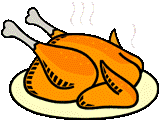## Food Science and Technology Department## Faculty Publications in Food Science and Technology

2020

#### Citation

AIMS Agriculture and Food, 5(4): 835–849. DOI: 10.3934/agrfood.2020.4.835

2020 the Author(s),

#### Abstract

This study examined the effect of collecting near infrared (NIR) spectra of forage samples through a transparent polypropylene (PP) plastic film instead of glass cups on calibrating two handheld NIR spectrometers to nitrogen content (N). The first device was a transportable spectrometer (H1) covering 790–2500 nm at 1 nm interval, while the second device was a smartphone spectrometer (H2) covering 900–1700 nm at 4 nm interval. The spectra from each spectrometer were subjected to principal component analysis (PCA) to identify wavebands for PP packaging that would interfere in subsequent partial least squares (PLS) regression modeling to predict N. PCA results showed that the loadings of the first principal component (PC1) of the first derivative of the spectra from H1 and loadings of the second principal component (PC2) of the second derivative of the spectra from H2 were useful in identifying wavebands due to PP film. Regression models for H1 had better prediction performance when spectra were collected through glass than through PP films, in terms of coefficient of determination (r2 = 0.958), standard error of prediction (SEP = 0.96 g kg−1), and ratio of performance to deviation (RPD) = 4.93 vs. (r2 = 0.942, −1 SEP = 1.13 g kg , and RPD = 4.17). Similar results were obtained for H2 using spectra collected 2 −1 2 through glass (r = 0.821, SEP = 1.73 g kg , and RPD = 2.72) than through PP (r = 0.499, SEP = −1 2.99 g kg , and RPD = 1.57). Removing peaks due to PP in the sample spectra improved the PLS 2 −1 2 modelsforH1(r =0.959,SEP=0.94gkg ,andRPD=5.02),butnotforH2(r =0.521,SEP= −1 3.17gkg ,andRPD=1.49).Hence, scanning samples through PP film scan reduce the accuracy of predicting N, but for some handheld NIR spectrometers, this could be overcome by excluding wavebands due to PP.

COinS